# Summer Addition and Subtraction Worksheets

Addition and subtraction worksheets for preschool children (Great Summer Practice)! Looking for preschool worksheets to help your students practice basic math skills this summer? We’ve got you covered!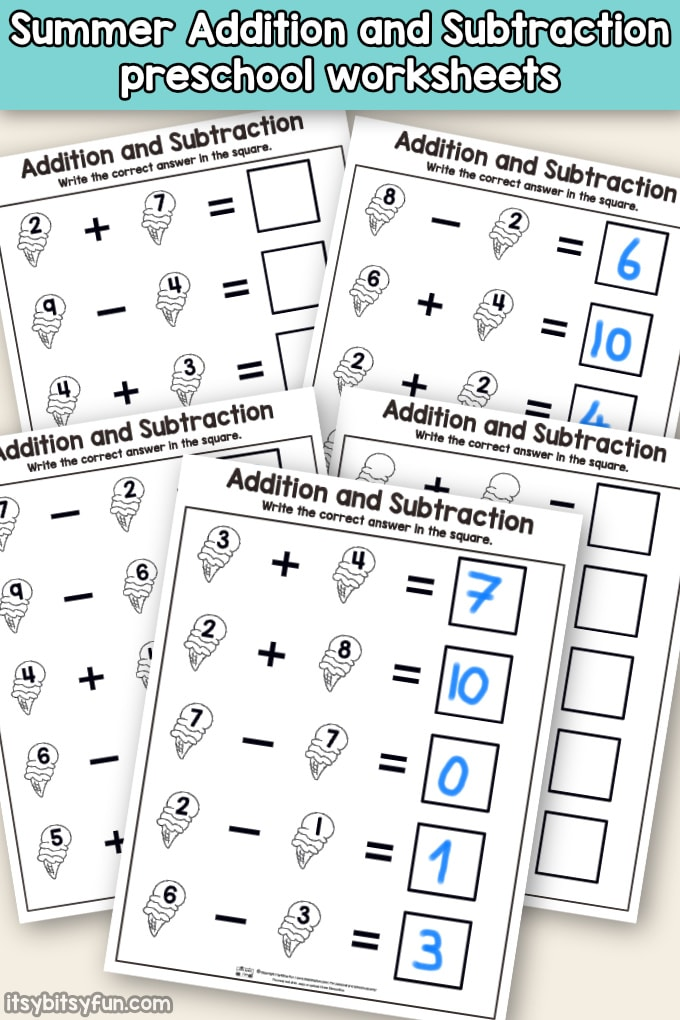These preschool math worksheets are designed to help young children practice addition and subtraction during the summer months.

Perfect for teachers and parents to use with preschool and kindergarten aged children, these preschool worksheets use whimsical images of ice cream cones to capture children’s imaginations.

Each worksheet contains five problems. Each equation is limited to numbers 1-10, and answers allow students to practice numbers up to 10. Students will solve the equations and write down the total in the box at the end of each row.

## Preschool Math Worksheets – addition and subtraction

Instead of separating addition worksheets from subtraction worksheets, we have mixed addition and subtraction problems in the same worksheets.

This added level of challenge helps increase flexibility in problem-solving.

However, you can reduce complexity by asking students to answer only the addition problems or only the subtraction problems.

We have also included a template worksheet on which you can write your own number combinations.

These addition and subtraction worksheets print on regular letter size (8.5 x 11 inch) computer paper and can be easily duplicated.

However, you can create a set of reusable worksheets by laminating the paper and using erasable markers or whiteboard markers.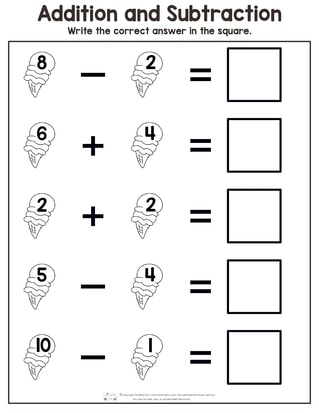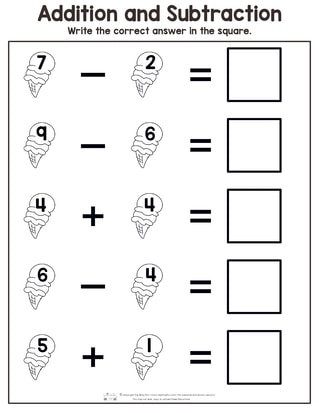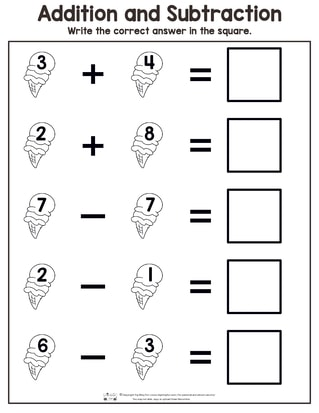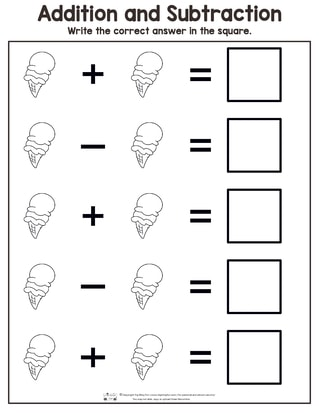If your students respond to games or competition, try challenging them by asking them to complete the worksheet within a certain amount of time.

You can also create a mini math tournament by dividing the class into paired brackets and having the winner of each bracket advance.

Winners could be determined based on the number of correct answers or the fastest time to completion.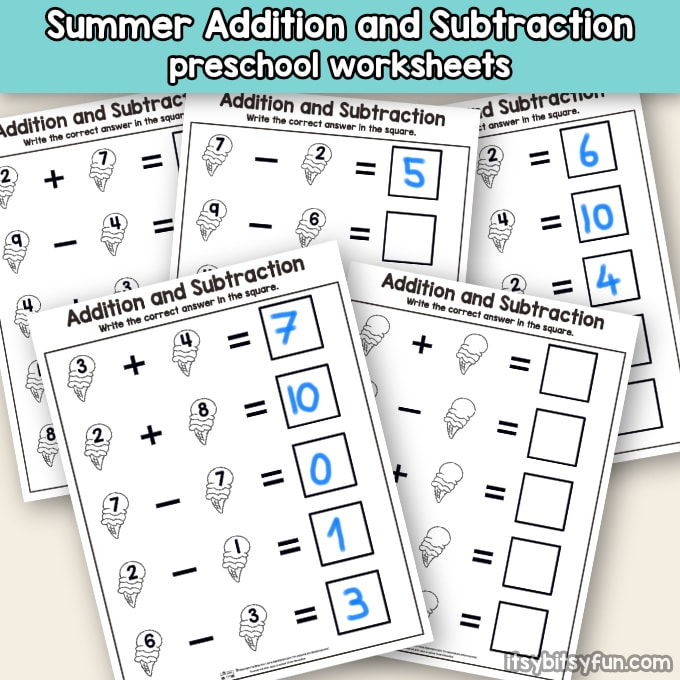Get your students ready for fall by keeping their math skills fresh over the summer!